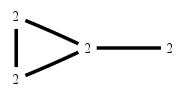# The Markov Bases Database

## G15g_bin

The binary graphical model of G15.

It is a hierarchical model of 4 variables. The dimension of the model is 9.The cardinality of the statespace is 16.

### Properties of the Markov basis

 Markov degree 2 12

 degree 2 12

The model has the following properties:

• All variables are binary.
• It is a graphical model.
• The minimal Markov basis is unique.
• The semigroup is normal.### FILES

 Markov basis: sufficient statistics matrix: G15g_bin.mar (594 b) G15g_bin.mat (402 b) G15g_bin.mod (27 b) G15g_bin.tar.gz (3.51 kb)